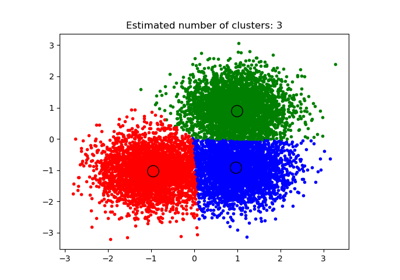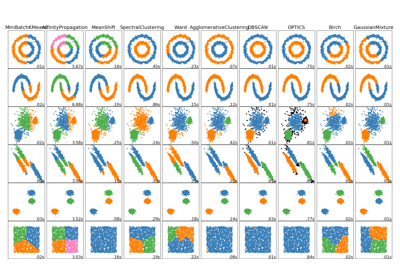# sklearn.cluster.estimate_bandwidth¶

sklearn.cluster.estimate_bandwidth(X, quantile=0.3, n_samples=None, random_state=0, n_jobs=None)[source]

Estimate the bandwidth to use with the mean-shift algorithm.

That this function takes time at least quadratic in n_samples. For large datasets, it’s wise to set that parameter to a small value.

Parameters: X : array-like, shape=[n_samples, n_features] Input points. quantile : float, default 0.3 should be between [0, 1] 0.5 means that the median of all pairwise distances is used. n_samples : int, optional The number of samples to use. If not given, all samples are used. random_state : int, RandomState instance or None (default) The generator used to randomly select the samples from input points for bandwidth estimation. Use an int to make the randomness deterministic. See Glossary. n_jobs : int or None, optional (default=None) The number of parallel jobs to run for neighbors search. None means 1 unless in a joblib.parallel_backend context. -1 means using all processors. See Glossary for more details. bandwidth : float The bandwidth parameter.

## Examples using sklearn.cluster.estimate_bandwidth¶A demo of the mean-shift clustering algorithmComparing different clustering algorithms on toy datasets